# How to Get Last Day of Month – Excel & Google Sheets

This tutorial will demonstrate how to get the last day of a month in Excel and Google Sheets.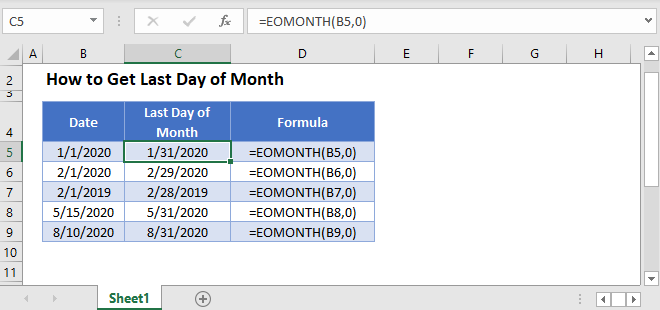## Get Last Day of Month

With the EOMONTH Function it is really straightforward to calculate the last day of a month.

``=EOMONTH(B3,0)``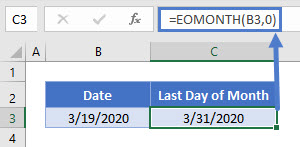The syntax of the EOMONTH function is EOMONTH(start_date, months). The ‘months’ argument specifies the number of months before or after the start_date.

So if you need the last day of next month, you can simply use:

``=EOMONTH(B3,1)``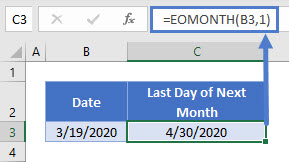You can also easily display the last day of last month like this:

``=EOMONTH(B3,-1)``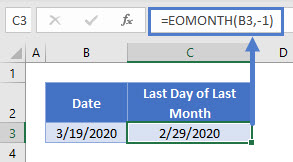## Last Day of Month – Text

Instead of displaying the last day of the month as a standard date, you can display it as text, using the TEXT Function.

``="The last day of the month is " & TEXT(EOMONTH(B3,0),"mm/dd/yyyy")``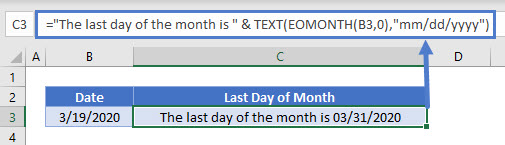Notice here, we’ve specified the date format of “mm/dd/yyyy”. There are many other date formats available to you.

## List Last Days of Month

You can even create a list of the last days of months with a given starting date.

``=EOMONTH(B3,C3)``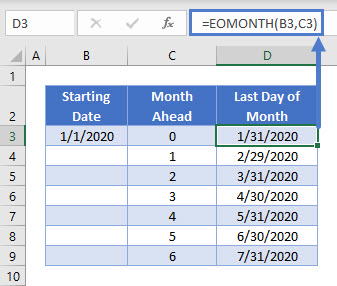## How to Get Last Day of Month in Google Sheets

These formulas work exactly the same in Google Sheets as in Excel.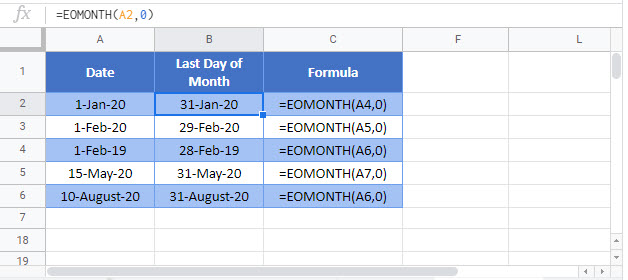### Excel Practice Worksheet

Practice Excel functions and formulas with our 100% free practice worksheets!